## Indicators

•Cited by SciELO
•Access statistics

•Cited by Google
•Similars in SciELO
•Similars in Google

## Print version ISSN 0012-7353

### Dyna rev.fac.nac.minas vol.82 no.192 Medellín July/Aug. 2015

#### http://dx.doi.org/10.15446/dyna.v82n192.48585

DOI: http://dx.doi.org/10.15446/dyna.v82n192.48585

An epidemiological approach to assess risk factors and current distortion incidence on distribution networks

Aproximación epidemiológica para evaluar factores de riesgo e incidencia de distorsión armónica en redes de distribución

Miguel Fernando Romero a, Luis Eduardo Gallego b & Fabio Andrés Pavas c

a Grupo de investigación PAAS-UN, Universidad Nacional de Colombia, Bogotá, Colombia. mfromerol@unal.edu.co
b Grupo de investigación PAAS-UN, Universidad Nacional de Colombia, Bogotá, Colombia. lgallegov@unal.edu.co
c Grupo de investigación PAAS-UN, Universidad Nacional de Colombia, Bogotá, Colombia. fapavasm@unal.edu.co

Received: April 29th, 2014. Received in revised form: February 21th, 2015. Accepted: July 14nd, 2015.

Abstract
A methodology that is based on epidemiological analysis to assess risk factors and harmonic distortion incidence rate in a distribution network is proposed in this paper. The methodology analyzes the current harmonics emission risk at the PCC due to the connection of disturbing loads. These loads are modeled, and multiple loads connection scenarios are simulated using Monte Carlo Algorithms. From the simulation results, potential risk factors for critical harmonics indicators are identified, leading to a classification of the scenarios into groups of exposed or unexposed to risk factors. Finally, the incidence rate of harmonics is calculated for each load connection scenario and the risk of critical harmonics scenarios due to the exposure to risk factors is estimated.

Keywords: Power Quality (PQ); harmonic distortion; risk factors; relative risk (RR).

Resumen
En este artículo se propone una metodología basada en el análisis epidemiológico para evaluar los factores de riesgo y la incidencia de distorsión armónica en una red de distribución. La metodología analiza el riesgo de emisión de corrientes armónicas en el PCC debido a la conexión de cargas perturbadoras. Dichas cargas se modelan y múltiples escenarios de conexión de cargas se simulan usando algoritmos de Monte Carlo. Posteriormente de las simulaciones se identifican los potenciales factores de riesgo para indicadores críticos de armónicos. Esto Permite clasificar los escenarios en grupos de expuestos y no expuestos a factores de riesgo. Finalmente, se calcula la tasa de incidencia de armónicos para cada escenario de conexión de carga y se estima el riesgo de escenarios críticos de armónicos debido a la exposición al factor de riesgo.

Palabras clave: Calidad de potencia; distorsión armónica; factores de riesgo, riesgo relativo.

1. Introduction

Currently, distribution systems are gradually moving to smart grids. The deployment of smart grids, including distributed energy resources, can reduce the cost of new power plants; facilitate carbon-oxide reduction; provide reactive power compensation, more flexible frequency regulation, and enhance the system security . However, some undesired effects such as unbalance, voltage fluctuation, and harmonics due to the performance of distributed energy technologies and nonlinear loads, could affect the power quality conditions in the grid. Critical harmonics scenarios are of a greater concern to engineers because they can overheat the building wiring, overheat transformer units, and cause protections tripping as well as random end-user equipment failures . Additionally, current harmonics conditions can become critical due to the connection of new electronic technologies like Compact Fluorescent Lamps (CFL), Electric Vehicles (EV), and Distributed Generation (DG), among others. The analysis of harmonics is essential to determine the performance and designing of the equipment, so as the optimal location for harmonic compensating devices.

Measurement systems and methodologies for power quality disturbances assessment are integrated to smart grids. In Colombia the assessment of power quality has been integrated to the design of smart grids through the research project SILICE III (Smart Cities: Design of a smart micro-grid). One of the aims of this project is to develop methodologies for evaluating potential emissions of harmonics due to the connection of new loads in a distribution system.

Several methodologies for evaluating harmonics have been proposed in the last decades. In  an assessment of harmonics at the point of common coupling, using the principle of filtering was proposed, which determined the customer dominant current harmonics. The use of indices for assessing continuous and discrete disturbances is proposed in , with the aim of identifying power quality problems in a 50 customer real system (20kV/400V). In , power quality indices throughout the system are analyzed. A measurement system consisting of a power quality disturbances monitoring system, a displaying platform, and diagnostic methodologies for the recorded information were proposed in this paper. Finally, in , a monitoring system with 250 computers by a Brazilian company is implemented. The system records the power quality disturbances and performs statistical analysis, estimating indicators and disturbances trends.

Since the last decade the impact of CFL on the harmonic levels in the network has been extensively studied. In  the impact of CFL on power quality in terms of total current and voltage harmonic distortion is evaluated. In  the effect of compact lamps during connection and disconnection to the distribution system is analyzed, then results of harmonics for cases of compact lamps and incandescent bulbs are compared. Finally, in the last five years, the impact of loads such as electric vehicles on power quality has been of particular interest because of future overcrowding. In  the potential impact on power quality due to the connection of electric vehicle chargers in existing distribution networks is evaluated and  analyze the potential impacts of several penetration levels of electric vehicle chargers on a test distribution network.

Conventional studies of power quality in distribution systems consist of statistical assessments of disturbances in each bus-bar and the calculation of global indicators that characterize the power quality of the system. Moreover, with the introduction of new technologies such as CFLs, EVs and DG the current conditions of power quality may vary in the future. Accordingly, the power quality assessment faces the following problems: (i) evaluation of the overall impact on power quality due to the massive penetration of new electronic loads, (ii) prediction of future power quality problems in order to implement possible solutions and (iii) the determining of the relationship between impact factors (topological location, connection of other users, types of loads, etc.) and power quality conditions. In order to find solutions for these problems, this paper proposes a methodology based on epidemiological theory in order to analyze harmonic conditions in the system with features of predictability and risk analysis.

In contrast to the previously cited studies, this paper conducts the harmonic evaluation based on epidemiological theory. This novel approach allows the risk of future critical harmonics scenarios to be evaluated due to the connection of new electronic loads and it determines their impact on system power quality conditions using epidemiological techniques. Specifically, the occurrence of a disturbance and its spread in the network is considered similar with the occurrence and spread of a human virus, spam, news, etc. Thus, epidemiology techniques can be a useful tool to assess power quality conditions for the estimation of risk indices to assess possible future harmonics conditions based on current conditions.

The paper is organized as follows: First, the problem of power quality is formulated from the epidemiological perspective. A characterization and modeling of disturbing loads is then performed through EMTP-ATP and MATLAB® software. Subsequently, several scenarios of load connections are proposed and stochastic simulations are performed in a real system model. The relationship between risk factors and incidence of harmonics in the simulated scenarios is estimated through risk indices. Finally, the risk of critical harmonics scenarios is calculated.

2. Epidemiological assessment of Power Quality

Epidemiological analysis has been used in several fields mostly related to biology, microbiology, and medical sciences [11-13]. However, there are several examples of its application in engineering which are related to the characterization of the epidemic spread of viruses on networks Internet, Wi-Fi, cell or local networks [14-17].

Conventional epidemiological methodologies try to answer the following questions in order to assess the health of a population:

• Who is at risk of becoming sick or infected?
• What is the rate of recovered and deceased patients?
• What factors affect the rate of infected population?

Similar to an epidemiological problem, a power quality problem tries to solve the following questions:

• What are the power quality conditions of the customers? (Health conditions)
• What is the number of customers who are affected or will be affected by power quality disturbances above a threshold or reference? (Rate of infected population)
• Which factors influence the occurrence of disturbances in these customers? (Risk factors)

In order to resolve these questions, epidemiological methodologies can be extended to power quality problems. Epidemiological assessment consists of a three-step process, as shown in Fig. 1.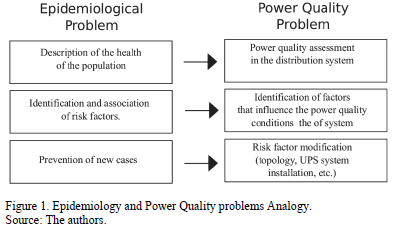A detailed explanation of each stage is given in the following sections.

2.1. Description of population health

Epidemiology observes phenomena related to diseases causing death in certain populations. These epidemiological phenomena are measured by means of two different and complementary ways: the prevalence ratio and the incidence ratio. The prevalence ratio (PR) describes the amount of existing cases of disease at a particular point in time. The incidence ratio (IR) studies the number of new cases of a disease within a specific period of time. According to reference , (PR) and (IR) are calculated as follows: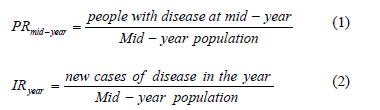In the equations (1) and (2) the periods of time of mid-year for prevalence ratio and year for incidence ratio are selected depending on the disease under study. A year is a common period of time for many epidemiological studies . Unlike most epidemiological studies, the power quality population remains constant and cause-effect temporality is instantaneous. Therefore, the period of time to assess the risk of critical disturbances is not considered on a power quality context and the prevalence ratio is equal to the incidence ratio. In order to assess critical power quality disturbances the incidence ratio (IR) on equation (3) is proposed.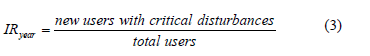In this paper, users with critical disturbances are defined as users with TDD levels above a reference value. The reference is taken from IEEE according to maximum demand load current and maximum short circuit current at PCC.

2.2. Risk/protective factors association

In this step epidemiology analyses the relationship between critical levels of disturbances and Risk/Protective factors. Risk factors contribute to the occurrence of the disturbance. Therefore, the greater the exposure of customers to risk factors, the higher the incidence of disturbance. Moreover, protective factors contribute to reducing the occurrence of the disturbance, thus, the greater the exposure of customers to protective factors, the lower the incidence of the disturbance.

Risk/protective factors could be identified as follows:

1. Formulation of a possible factor that could affect the incidence of the disturbance.
2. Establishing two groups of customers characterized by their exposure (exposed and non-exposed) to a possible risk/prevention factor.
3. Measurement of the incidence of the exposed and non-exposed groups.
4. Measurement of the association based on the values indicating the relationship between the presence of the factor and the occurrence of the disturbance.

The measurement of the association is the difference of the disease frequency between the exposed and the non-exposed group. This measurement is achieved through the following risk indicators:

• Relative Risk (RR)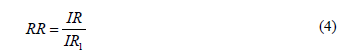• Attributable Risk (AR)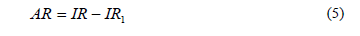• Proportion of Exposed Attributable Risk (PEAR)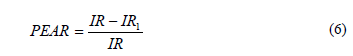Where:

IR is the incidence ratio on exposed users, and
IR1 is the incidence ratio on unexposed users.

The analysis of the above risk indicators shows the relationship between risk factors and incidence ratio of the disturbance. The strength of the identified relationship depends on the values of those indicators.

The particular case of IR1=0 means that there are no other risk factors that could cause critical disturbances in the user. Therefore the possibility of a critical scenarios occurrence is attributed entirely to the risk factor under study. However, several risk factors (Short circuit level, disturbing neighbor loads, etc.) could affect power quality conditions in real scenarios, thus IR1=0 is unlikely. The interpretation of each indicator on equations (4), (5) and (6) in the context of power quality will be described in more detail in the next sections.

2.3. Prevention of new cases

After identifying the risk factors that directly or indirectly affect the incidence of a disturbance, the epidemiological methodology assesses the corresponding actions to reduce the exposure to these factors.

3. Definition of risk factors, environment, and population

With regard to the power quality issues, in this paper the risk of reaching critical harmonics levels is analyzed at the PCC, as depicted in Fig. 2.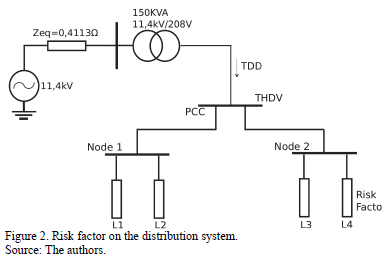The circuit in Fig. 2 is a real 11,4kV/208V feeder at the National University of Colombia. In order to make a precise feeder modeling, the following parameters were considered:

• Network Equivalent. A network equivalent represents the feeder voltage variations characteristics and the impact of power quality disturbances at the upstream grid. In order to model the distribution network equivalent, the short circuit impedance 0.4113W; was obtained from the network operator.
• Distribution Lines. The model considers the cable type (overhead or underground), the line caliber and physical characteristics proper of each facility.
• Transformers. A model was developed, representing all the parameters of the transformer by using the available manufacturer data.

The total demand distortion index (TDD) in equation (7) is used to assess the impact of risk factor (L4) on harmonic distortion at the PCC .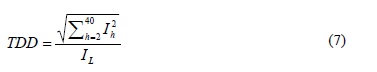Where:

Ih= Harmonic current component in amperes with the harmonic order indicated by the subscript h.
IL= Maximum demand load current in amperes (fundamental frequency component) at PCC.

The current distortion limit of TDD is used as a reference to determine which scenarios of harmonics distortion are considered to be critical. Table 1 shows the limits values for current distortion for general distribution systems according to IEEE 519 .In the case of the circuit in Fig. 2, the relationship between (Isc) and (IL) is 192.3. According to Table 1, the reference value to assess TDD indices at the PCC is TDD=15%.

In this case, a critical harmonics level is defined as the value of total demand distortion TDD, measured at the PCC over the reference level. When the point of the system has waveform distortion levels above the reference, it is considered to be a critical point.

3.1. Risk factors hypothesis

Several factors could affect the harmonics incidence in a point of the network. Some of these factors are:

• Short circuit level. Although short circuit level is not a direct cause of harmonics disturbances in a network point, this parameter could favour the presence of harmonics due to other causes.
• Linear and non-linear loads on customers. Connection of several types of loads can cause high harmonics levels at the PCC.
• Harmonics disturbances from other circuits. Loads connected to other nearby circuits could affect the harmonics level in the current feeder.
• Capacitor banks used for voltage control and power factor improvement. The connection of capacitors can cause resonance conditions that can affect harmonics levels.

In this paper the connection of non-linear loads at user L4 is analyzed as a risk factor for the harmonics incidence at the PCC.

3.2. Environment and population

In order to analyze the relationship between the risk factor and the current harmonics incidence at the PCC, individuals belonging to a population are defined based on the circuit in Fig. 2. L1, L2, and L3 represent linear and non-linear loads that users can connect at nodes 1 and 2. L4 is a non-linear load and represents the risk factor of interest. Linear loads are modeled by equivalents RL with RMS currents between 0A and 50A. Non-linear loads are modeled like full wave rectifiers with capacitive filter and a variable resistance with RMS currents between 0A and 50A.

Every individual in the population is represented by every possible load connection scenario in the circuit. In order to generate the study population and risk factors levels, several connection scenarios are proposed as follows:

1. Generating study population. 169 different load scenarios of L1, L2, and L3 are generated through current variations of each load between 0A and 50A. An example of loads combination in order to obtain a view of the load scenarios is shown in Table 2.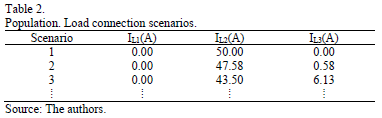1. Generating risk factor levels. 12 levels of the risk factor L4 are generated by means of current variation of the load between 0A and 50A. An example of risk factor levels is shown in Table 3.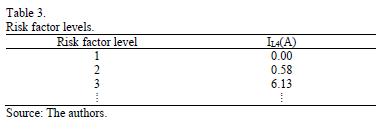1. Stochastic simulation. Load scenarios are generated by combining L1, L2, L3, and risk factor L4 vectors. Load combinations are included to observe the cumulative effect of loads on the harmonics level. Finally 2028 cases were obtained by simulating 169 scenarios for each 12 risk factor levels.

Harmonics levels at PCC in all scenarios are obtained by simulations in time domain. The results are classified and analyzed in the following section in order to determine the association between risk factors and the level of harmonics.

In the following sections an epidemiological methodology is used to assess several scenarios of harmonics levels in the distribution network.

4. Assessment of association levels due to harmonic disturbances in distribution networks

In this section the above explained epidemiological analysis is applied to previous simulations. First, an evaluation of the power quality status of the entire study population (load connection scenarios) is performed. Subsequently a reference TDD value is proposed to evaluate the population in terms of "sick" and "healthy". Finally the population is classified into exposed and unexposed to the risk factor and risk indicators are calculated.

4.1. Description of the epidemiological power quality status

The study population consists of 169 different load scenarios or individuals. Every individual is exposed to 12 different levels of the risk factor. Therefore, the total population contains 2028 individuals or load scenarios.

Every load scenario is simulated on ATP-EMTP software computing current and voltage signals. These signals are processed to calculate the electrical variables and the harmonic levels at the PCC.

Table 4 shows the statistical indicators of voltage, current, and harmonic distortion observed at the PCC for the 2028 load connection scenarios.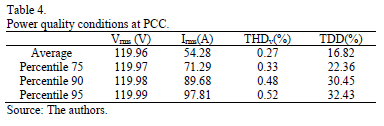From Table 4 the average voltage distortion THDv is about 0.27%, which is very low according to the reference value in IEEE 519 standard (5%).

Furthermore, the average total demand distortion TDD is 16%. According to Table 1 these values exceed the TDD limits (15%). Therefore, the possibility of critical harmonic levels due to the loads connection could be very high. In the next paragraph, the epidemiological methodology is applied to estimate the impact of connecting L4 load on the TDD level observed at the PCC.

4.2. Risk factor identification and association

According to Fig. 3, individuals of the population are split into two groups, exposed and unexposed to the risk factor. Unexposed individuals are the load scenarios where risk factor L4 is not connected. On the other hand, exposed individuals are load scenarios where L4 is connected. Subsequently, a reference value of current distortion TDD=15% is defined and individuals with critical conditions of current distortion are identified in exposed and unexposed groups.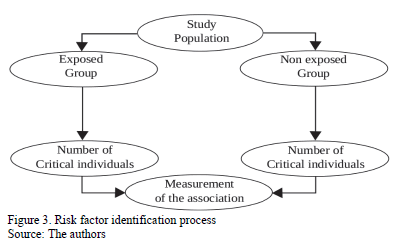Furthermore, as L4 could have different current load conditions, the risk factor can have several levels of exposure. In order to verify the individual impact of each of these 12 exposure levels in the occurrence of critical harmonic distortion, every level is considered as an exposed group and the incidence is calculated for each of them. Table 5 shows incidence values for 12 different exposure levels of the risk factor. Level 1* indicates the particular unexposed case.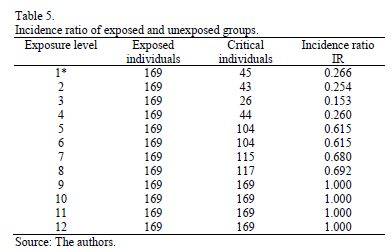According to Table 5, the unexposed group 1* has 169 individuals, from which 45 have TDD values above the threshold (critical individuals). Therefore, the incidence ratio of high harmonics in the population according to equation (2) is: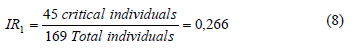In the case of exposure level 8, the incidence ratio of critical distortion is calculated as follow: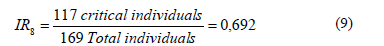The Fig. 4 shows the average and 95 percentile values of TDD(%) for each exposure level.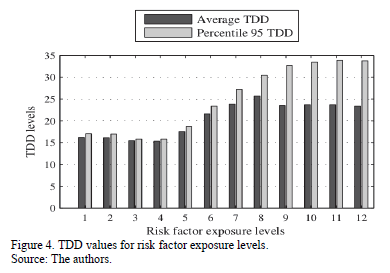From Fig. 4, level 1* (unexposed) has a TDD average value around 16.18%. This current distortion is caused by the presence of others nonlinear loads different than L4 (risk factor). For exposure levels between 2 and 4, the TDD average decreases from 16.14% to 15.35%. For exposure levels between 4 and 12, the TDD average increases from 15.35% to 23.36%.

In order to analyze the association between levels of exposure to the risk factor and the presence of TDD critical scenarios, attributable risk (AR), relative risk (RR) and proportion of exposed attributable risk (PEAR) are calculated from equations (5), (4), and (6). The results for each exposure level are shown in Table 6.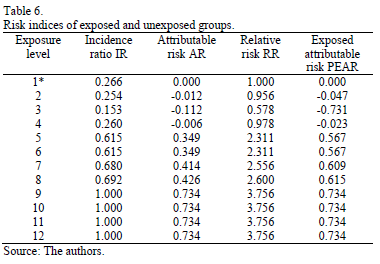Fig. 5 shows the incidence of critical TDD scenarios (IR) and attributable risk (AR) that are calculated according to equation (5). The attributable risk is the portion of incidence that is due to exposure to the risk factor. The interpretation of the attributable risk is as follows: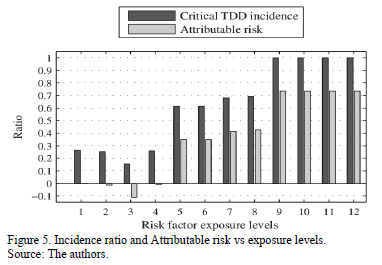In the case of a risk factor level equal to 5, the incidence ratio of critical TDD scenarios is 0.615 and the attributable risk is 0.349. This means that 0.349 of 0.615 is due to the risk factor under study.

In Fig. 6, the relative risk measures how big the impact of the risk factor is as compared with the unexposed scenario to that factor. Fig. 6 shows the relative risk for each exposure levels.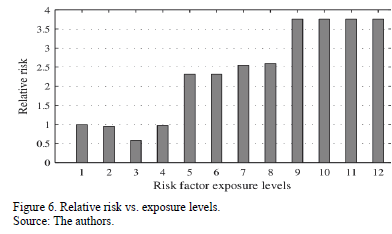The interpretation of the relative risk is as follows:

In the case of a risk factor level equal to 5, the risk of critical TDD scenarios increases 2.311 times as compared with the unexposed scenario.

Fig.7 shows the exposed attributable risk. This indicator shows the proportion of risk that is due to exposure to the factor under study. In the case of level 5, the following interpretation holds: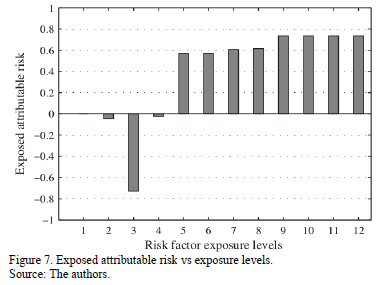In the case of a risk factor level equal to 5, the 56.7% of critical TDD scenarios are due to factor exposure. The rest of the risk is due to the presence of other factors.

For the particular case of exposure level 3, the factor L4 is considered protective of 73.1% of the occurrence of critical TDD scenarios.

According to Fig. 5 and 6, the impact depends on the level of exposure to the risk factor; it therefore depends on the current level of the disturbing load L4. Due to the presence of harmonics induced by other loads, the risk factor incidence could interact with them and the result of this interaction is the TDD levels on the main feeder. An example of this is level 4, wherein the interaction between the risk factor with other disturbances decreases the risk of critical TDD levels on the feeder. In this case, the interaction with L4 acts as a protective factor. Moreover, in cases 5 to 12, the interaction of L4 increases the risk of high TDD levels, thus L4 acts as a risk factor and the higher the current, the greater the risk of critical TDD scenarios.

4.2. Prevention of new cases

In order to prevent or reduce the risk of high TDD levels two possibilities are proposed. First, the exposure to the risk factor could be reduced. In this case, scenarios 5 to 12, where the risk of high TDD levels increases significantly, need to be avoided. Another option is to connect loads whose interaction with the present loads decreases the risk of TDD values above desired limits. This methodology can be extended to analyze complex load interactions. From this perspective, epidemiology offers tools to verify the interaction between variables and to evaluate complex problems of power quality with a new approach.

5. Conclusions

A methodology based on epidemiological analysis for assessing risk factors and incidence rates of harmonic distortion in a distribution network was proposed. Current harmonics emission risks at the PCC due to the connection of disturbing loads were analyzed. Multiple loads connection scenarios were simulated using Monte Carlo Algorithms. From the simulation results, potential risk factors for critical harmonics indicators were identified and connection loads scenarios are classified into exposed and non-exposed to these risk factors. Finally, the incidence ratio of harmonics, the attributable risk, the relative risk and exposed attributable risk were calculated, allowing the identification of critical connection loads scenarios. The results show an interesting application of epidemiological methods in characterizing the grid vulnerability under harmonic pollution and the proposed methodology may be easily extended to other types of power quality disturbances.

Acknowledgements

The authors would like to thank to COLCIENCIAS, CODENSA S.A. E.S.P., the National University of Colombia and PAAS-UN Research Group for the support given during the execution of the research project SILICE III (Smart Cities: Design of a smart micro-grid).

References

 Su, H.J., Chang, G.W., Ranade, S. and Lu, H.J., Modeling and simulation of an AC microgrid for harmonics study, Harmonics and Quality of Power (ICHQP), 2012 IEEE 15th International Conference, pp.668-672, 17, 20 June, 2012.         [ Links ]

 Mikkili, S. and Panda, A.K., Mitigation of harmonics using fuzzy logic controlled shunt active power filter with different membership functions by instantaneous power theory. In Students Conference on Engineering and Systems. pp. 1-6, 2012. DOI: 10.1109/sces.2012.6199103        [ Links ]

 Huang, X., Nie, P. and Gong, H., A new assessment method of customer harmonic emission level. In Asia-Pacific Power and Energy Engineering Conference, pp. 1-5, 2010. DOI: 10.1109/appeec.2010.5449329        [ Links ]

 Siahkali, H., Power quality indexes for continue and discrete disturbances in a distribution area. In IEEE 2nd International Power and Energy Conference, pp. 678-683, 2008. DOI: 10.1109/pecon.2008.4762561        [ Links ]

 Chung, I.Y., Won, D.J., Kim, J.M., Ahn, S.J., Moon, S.I., Seo, J.C. and Choe, J.W., Development of power quality diagnosis system for power quality improvement. In Power Engineering Society General Meeting, IEEE, 2, pp. 1256-1261, 2003.         [ Links ]

 Mertens, E., Dias, L., Fernandes, E., Bonatto, B., Abreu, J. and Arango, H., Evaluation and trends of power quality indices in distribution system. In Electrical Power Quality and Utilization, EPQU 2007. 9th International Conference, pp. 1-6, 2007. DOI: 10.1109/epqu.2007.4424212        [ Links ]

 Jabbar, R. and Al-Dabbagh, M., Impact of compact fluorescent lamp on power quality. Power Engineering Transactions, pp. 1-5, 2008.         [ Links ]

 Matvoz, D., Impact of compact fluorescent lamps on the electric power network. Harmonics and Quality of Power, pp. 1-6, 2008. DOI: 10.1109/ichqp.2008.4668864        [ Links ]

 Putrus, G.A., Suwanapingkarl, P., Johnston, D., Bentley, E.C. and Narayana, M., Impact of electric vehicles on power distribution networks, IEEE Vehicle Power and Propulsion Conference, pp. 827-831, 2009. DOI: 10.1109/vppc.2009.5289760        [ Links ]

 Richardson, P. and Flynn, D., Impact assessment of varying penetrations of electric vehicles on low voltage distribution systems. Power and Energy Society, pp. 1-6, 2010. DOI: 10.1109/pes.2010.5589940        [ Links ]

 Britton, T., Stochastic epidemic models: A survey. Mathematical Biosciences. Elsevier Inc.Department of Mathematics, Stockholm University, SE-10691 Stockholm, Sweden. pp. 24-35, 2010.         [ Links ]

 Altowaijri, S., Mahmoud, R. and Williams, J., A quantitative model of grid systems performance in healthcare organizations. In 2010 International Conference on Intelligent Systems, Modelling and Simulation, pp. 431-436, 2010. DOI: 10.1109/ISMS.2010.84        [ Links ]

 Corley, C.D. and Mikler, A.R., A computational framework to study public health epidemiology. International Joint Conference on Bioinformatics, Systems Biology and Intelligent Computing, p 360-363, 2009. DOI: 10.1109/ijcbs.2009.83        [ Links ]

 Liu, Z. and Lee, D., Coping with instant messaging worms, statistical modeling and analysis. In 15th IEEE Workshop on Local & Metropolitan Area Networks, pp. 194-199, 2007. DOI: 10.1109/lanman.2007.4295998        [ Links ]

 Hashim, F. and Munasinghe, K.S. and Jamalipour, A., Biologically inspired anomaly detection and security control frameworks for complex heterogeneous networks, in: IEEE Transactions on Network and Service Management, pp. 268-281, 2010. DOI: 10.1109/tnsm.2010.1012.0360        [ Links ]

 Chao-Sheng, F., Zhi-Guang, Q. and Ding, Y., Modeling passive worm propagation in mobile P2P networks. In: International Conference on Communications, Circuits and Systems (ICCCAS), pp. 241-244. 2010. DOI: 10.1109/ICCCAS.2010.5582004        [ Links ]

 Bowei, S., The spread of malware on the WiFi network: Epidemiology model and behaviour evaluation, In: Information Science and Engineering (ICISE), 2009 1st International Conference, pp. 1916-1918, 2009.         [ Links ]

 Woodward, M., Epidemiology, study design and data analysis. Statistics in Medicine, 2nd ed., Vol. 25, 699P. Boca Raton, Chapman & Hall/CRC, 2005.         [ Links ]

 Norma Técnica Colombiana NTC 5001. Calidad de la potencia eléctrica. Límites y metodología de evaluación en punto de conexión común, ICONTEC. 2008.         [ Links ]

 IEEE Recommended practice and requirements for harmonic control in electric power systems, IEEE Std 519-2014, Revision of IEEE Std 519-1992, pp.1-29, 2014.         [ Links ]

M.F. Romero received his BSc. in 2006 and MSc. in 2011 in Electrical Engineering from the Universidad Nacional de Colombia, Bogotá, Colombia. Currently, he is developing a PhD in Electrical Engineering at the same institution. He has been a researcher in the Program of Acquisition and Analysis of Electromagnetic Signals - PAAS at the Universidad Nacional de Colombia - since 2005. His research interests include power quality, power systems analysis and network distribution design.

L.E. Gallego, received his BSc. in 1999, MSc. in 2002, and PhD in 2008 from the Universidad Nacional de Colombia, Bogotá Colombia. He has been a researcher in the Research Program of Acquisition and Analysis of Electromagnetic Signals of the Universidad Nacional de Colombia - PAAS-UN since 2000, working in research projects mainly related to power quality, lightning protection, and power markets. He is involved in teaching activities related to power quality and computational intelligence. His research interests include power quality analysis, power markets and computational intelligence applied to power systems modelling.

A. Pavas, received his BSc. in 2003) and MSc. in 2005 in Electrical Engineering from the Universidad Nacional de Colombia and finished his PhD in Electrical Engineering at the same institution. Pavas has been a researcher in the Program of Acquisition and Analysis of Electromagnetic Signals of the National University of Colombia-PAAS-UN Group since 2001, where he has proposed and executed research projects related to power quality and lightning protection. He is a lecturer in the Universidad Nacional de Colombia in the areas of electric machinery and power quality. His research interests include power quality, electromagnetic compatibility and probabilistic aspects of power quality.